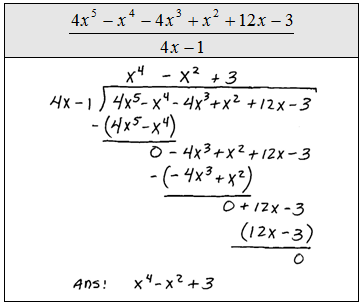## Algebra

### Dividing Polynomials

In this section we will learn how to divide polynomials. Students find this to be one of the more difficult topics in Algebra. Plan on spending some extra time reviewing the techniques and solutions presented here. (Assume all expressions that appear in a denominator are nonzero.)

Dividing by a Monomial
When dividing we will be using the quotient rule,This says that when dividing two expressions with the same base you subtract exponents.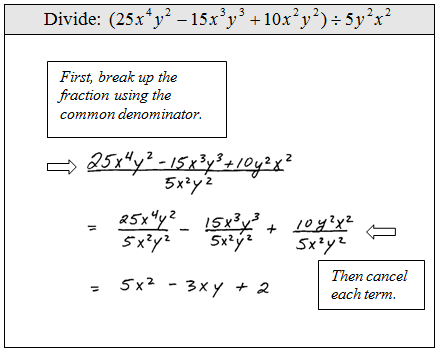In fact, we are using the property for adding fractions with a common denominatorBy breaking up the fraction we could then simply and then cancel.

Divide.A common mistake would be to cancel denominator with only one of the terms. We are dividing the entire expression in the numerator so every term must be cancelled with the denominator.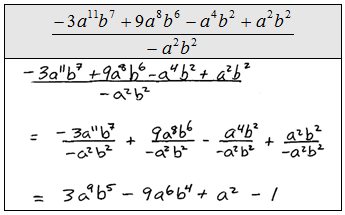Dividing by a Polynomial
In this section, we will use polynomial long division when dividing by something other than a monomial. The good news is that the steps are basically the same as the regular division algorithm we are already used to. Use polynomial long division to divide the following.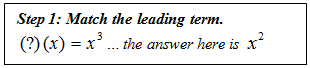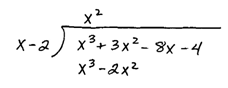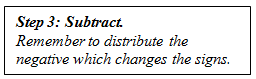The completed process follows.Division does not always work out so evenly, sometimes you will have a remainder.Divide.Some of the polynomials will be missing terms. In other words, not all the exponents will be there. When first learning, it really is best to use placeholders and include the missing terms using zero as coefficients.Divide.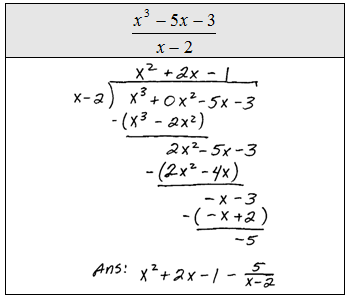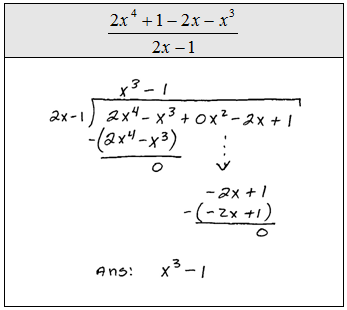Polynomial long division takes some practice to master. Be patient, do lots of problems and soon you will find them to be enjoyable.

Divide.